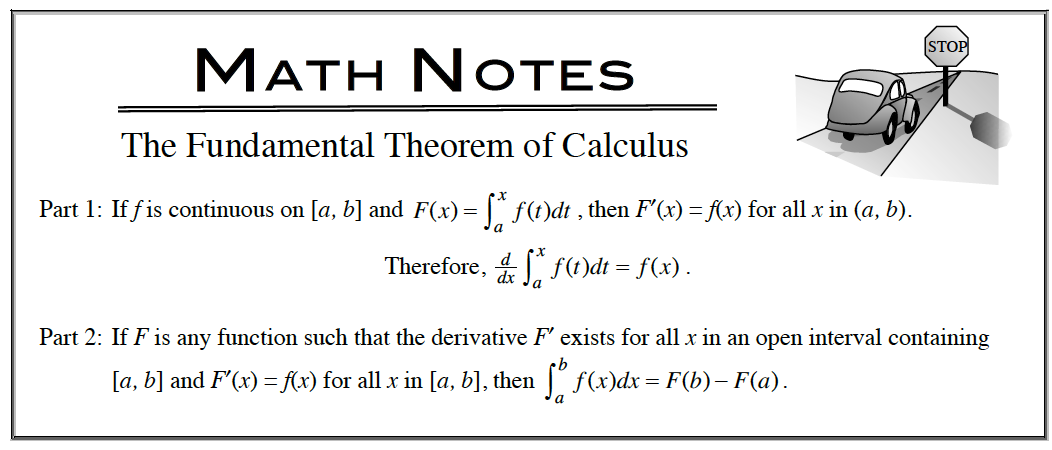### Home > APCALC > Chapter 5 > Lesson 5.2.1 > Problem5-51

5-51.

Evaluate the following integrals without a calculator. Homework Help ✎

1. $\int _ { 1 } ^ { 4 } ( 3 \sqrt { u } + \frac { 1 } { \sqrt { u } } ) d u$

Before integrating, rewrite the integrand with exponents.

2. $\int _ { 0 } ^ { 2 } 6 ( x - 4 ) ^ { 2 } d x$

Before integrating, expand the integrand.

3. $\int \frac { d } { d x } [ g ( x ) ] d x$

Part 1 of the Fundamental Theorem of Calculus states that the derivative of an integral equals the integrand. This problem asks you to find the integral of the derivative. How will the result differ?4. $\int _ { 0 } ^ { 1 } k ^ { 5 / 3 } d k$

$= \frac { k ^ { 8 } } { \frac { 8 } { 3 } } | _ { 0 } ^ { 1 } = \frac { 3 } { 8 } - 0 = \frac { 3 } { 8 }$

Evaluating this integral is not that difficult.
But are you using proper notation?

5. $\int _ { 1 } ^ { 2 } \frac { 4 } { n ^ { 3 } } d n$

Before integrating